# Write Your Own Functions in R – III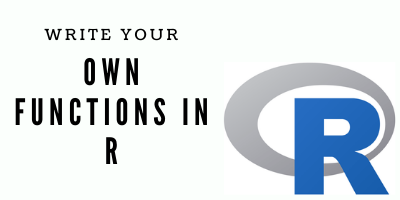Welcome to the third article of my series “Writing your own functions in R”. This weekend we will see how we can create attractive excel reports using openxlsx package.

In the previous article, we wrote a function that iterates through all “.xlsx” files stored within a folder and while scanning through individual files, it would print the name of the file, number of sheets present in it and the names of all sheets in the file. Finally, it would also generate a data frame storing all of the above information. In this article, we will use some of the functions in R from openxlsx package within a function that we will write to create some excel reports.

1. Learn how to write data frames stored in the R environment into files with the “.xlsx” extension, this is pretty simple.
2. Learn how to write multiple data frames into different sheets of a “.xlsx” file using functions from openxlsx This we will learn in two different ways.
3. Create custom styling for header and body for data frames to be written into excel files and applying them within a custom function.

Let us start by creating a random data frame. We shall use the function, expand.grid.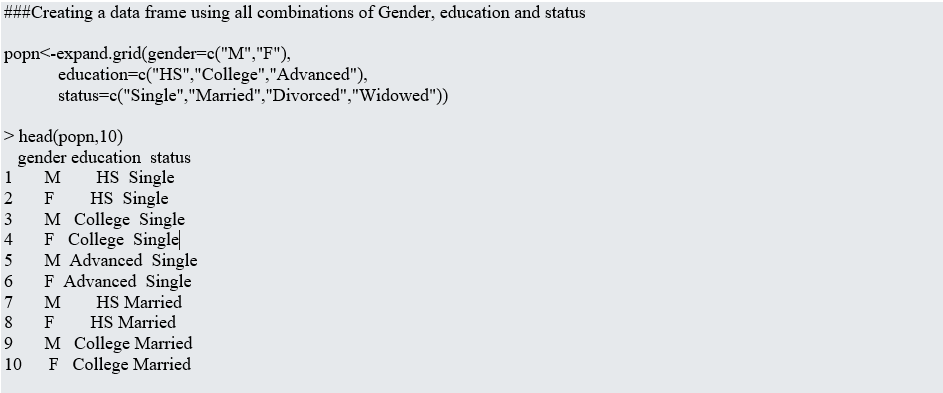Now, writing this data frame in an excel file is pretty simple, though there are two different ways of doing this. Let us see those below,

### Option 1

```
############Snippet 2##################
write.xlsx(popn,"popn.xlsx")

### Option 2 ###

### More Relevant while adding multiple sheets in an excel file ###

wb&lt;-createWorkbook() # Create an empty WorkBook

writeData(wb,"Sheet1",popn) # Write the Data Frame in the added Worksheet

saveWorkbook(wb,"popn.xlsx") # Save the WorkBook as an excel file.

```

Both the ways used above produce the same outputs, the advantage of using the second option over the first one is that we can add multiple sheets in an excel file using the later.

Let us now try adding multiple data frames in different sheets of an excel file. For that, we need to generate an additional data frame. We will do this in a similar fashion as before using expand.grid.

`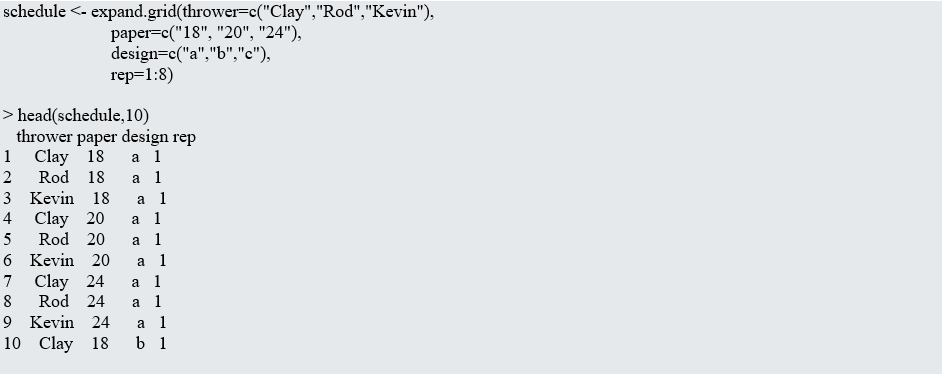`

Now simply using the first option as before we cannot add multiple sheets within the same excel workbook, for that, we will create a named list.

The names of elements within the list will be the names of sheets to be added in the workbook, while the elements will be the data frames we wish to add in those sheets. The other way around is similar to the second option we have seen above, however since that is a little repetitive we can use a loop to ease our task.

Let us see these below:

```
############################# Option 1 ###############################

list_of_data<- list("Population"=popn, "Schedule"= schedule)

write.xlsx(list_of_data,"Dataframes.xlsx")

############################ Option 2 ################################

wb<-createWorkbook() # Create Empty Work Book

writeData(wb,"Population",popn) # Add "popn" to WorkSheet 1

writeData(wb,"Schedule",schedule) # Add "schedule" to WorkSheet 2

saveWorkbook(wb,"Dataframes.xlsx") # Save WorkBook

```

The second way of doing this again looks pretty repetitive and manual. However, we can make it more efficient by writing a simple loop,

```
list_of_data<- list("Population"=popn, "Schedule"= schedule)

Z=0

wb<- createWorkbook()

for(i in 1:length(list_of_files)){
Z=Z+1
writeData(wb,Z,list_of_files[[Z]],withFilter = F)
setColWidths(wb, sheet = Z, cols = 1:ncol(list_of_files[[Z]]),
widths = "auto")
}

if(Z==length(list_of_files)){
suppressMessages(saveWorkbook(wb,file = "Dataframes.xlsx",overwrite = T))

}

######################### Create a Function out of it ###########################

write_files_xlsx<-function(list_files){
Z=0
wb<- createWorkbook()
for(i in 1:length(list_of_files)){
Z=Z+1
writeData(wb,Z,list_of_files[[Z]],withFilter = F)
setColWidths(wb, sheet = Z, cols = 1:ncol(list_of_files[[Z]]),
widths = "auto")
}
if(Z==length(list_of_files)){
suppressMessages(saveWorkbook(wb,file = "Dataframes.xlsx",overwrite = T))
message("Dataframe Generated!")
}

}

> write_files_xlsx<-function(list_files = list_of_files)
Dataframe Generated!

```

The excel file generated looks like this: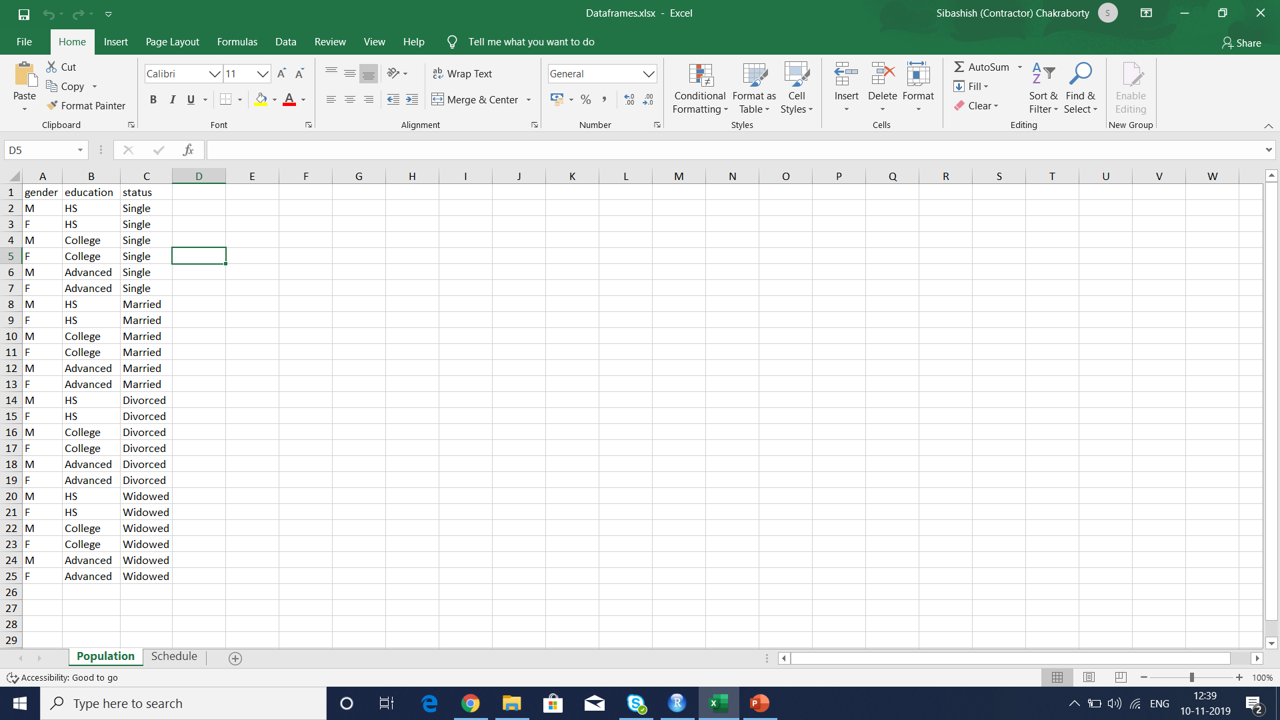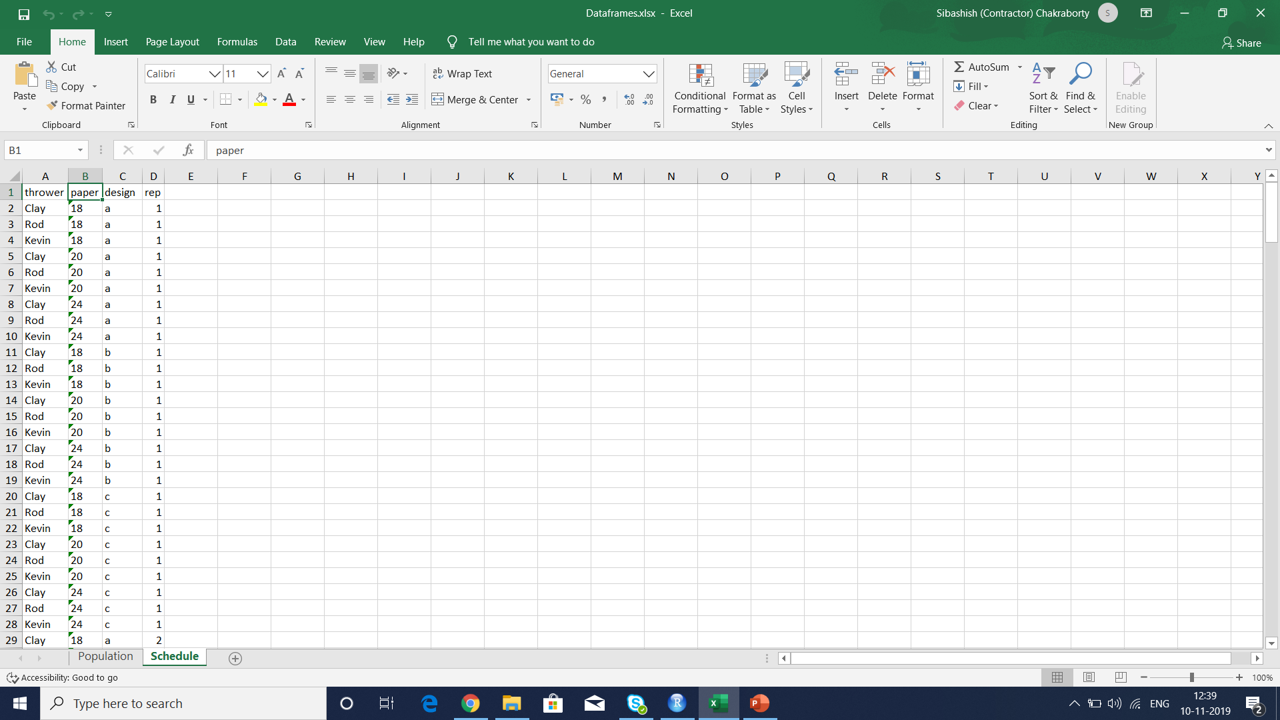These sheets look similar to normal excel sheets but without any formatting. But what if we would like to add a little more style to their appearance.

For example, I usually like the font size to be 9, the headers to be a little different so to distinguish from the body and probably add a thin border all around the cells.

All of this can be done in excel with a few simple steps and fortunately, this can be done even while generating these files from within R. All we need to do is add some “Style Statements” to our code and make the files look prettier.

Let us see those below,

```
############################# Create Styles #################################

mystyle<-createStyle(fontSize = 9,border = c("top", "bottom", "left", "right"),borderStyle = "thin",halign = "center",valign = "center")

headerStyle <- createStyle(fontSize = 9, fontColour = "#FFFFFF", halign = "center",
fgFill = "#4F81BD", border=c("top", "bottom", "left", "right"), borderColour = "#4F81BD", textDecoration = "bold")

################## Use Defined Styles inside the Function ###################

write_files_xlsx<-function(list_files){

Z=0

wb<- createWorkbook()

mystyle<-createStyle(fontSize = 9,border = c("top", "bottom", "left", "right"),borderStyle = "thin",halign = "center",valign = "center")

headerStyle <- createStyle(fontSize = 9, fontColour = "#FFFFFF", halign = "center", fgFill = "#4F81BD", border=c("top", "bottom", "left", "right"), borderColour = "#4F81BD", textDecoration = "bold")

for(i in 1:length(list_of_files)){
Z=Z+1
addStyle(wb,sheet = Z,mystyle,rows =1:nrow(list_of_files[[Z]])+1 ,cols = 1:ncol(list_of_files[[Z]]),gridExpand = T)
setColWidths(wb, sheet = Z, cols = 1:ncol(list_of_files[[Z]]),
widths = "auto")
}
if(Z==length(list_of_files)){
suppressMessages(saveWorkbook(wb,file = "Dataframes.xlsx",overwrite = T))
message("Dataframes Generated!")
}

}

> write_files_xlsx(list_files = list_of_files)
Dataframe Generated!

```

Let us take a look at the generated data frame again,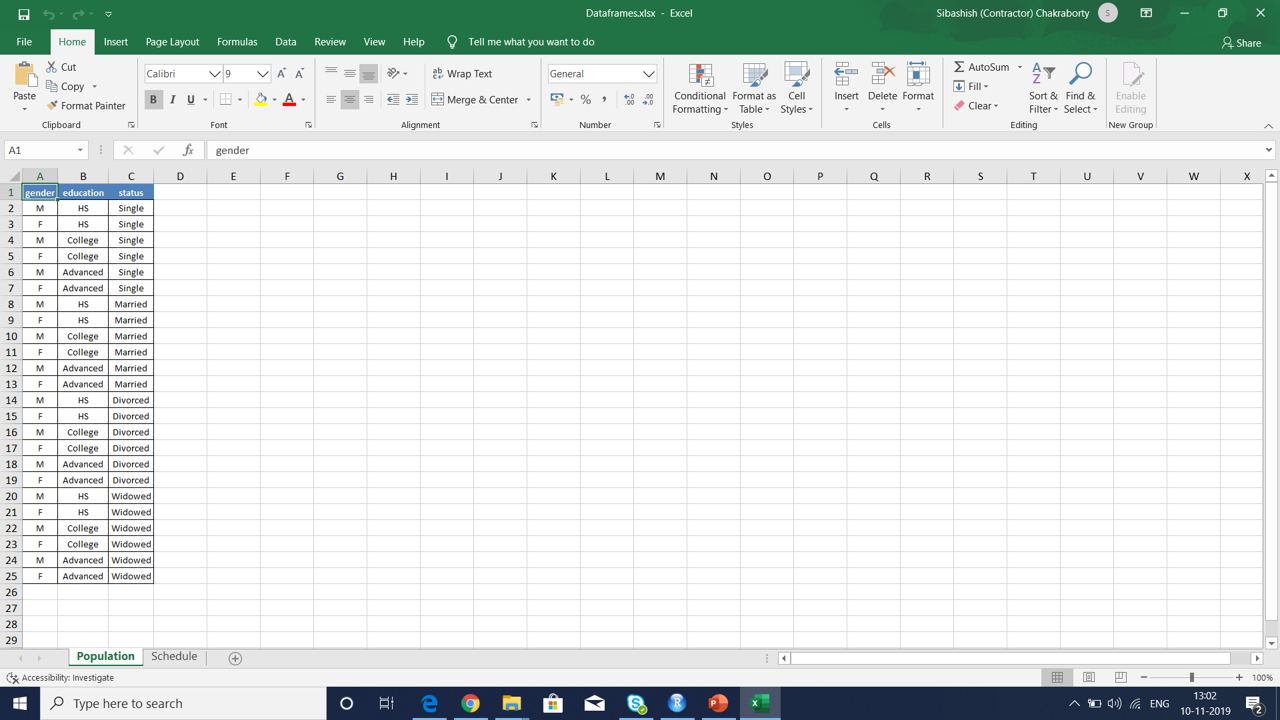Much better right? We can add many more such customization using different features within the package. For example, if I wanted the data to be formatted as a table, I could simply replace writeData() with writeDataTable().

Finally, we can integrate this together with other custom functions in R to make tasks easier for us. That is all for today’s article. See you all again in the next one! Till then.., Ciao!